# Binary Search Trees

### Trees

The data structures we have used so far have been linear by nature. Arrays, linked lists, stacks and queues all of a definite start and end. Today we will look at a type of data structure, the tree, where this is not the case.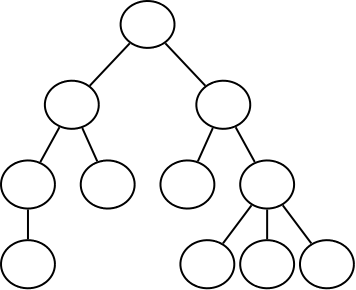Unlike organic trees, the tree data structure is normally drawn upside down. There are a few different terms used when discussing trees:

• Node: As with linked lists, a node is simply one element of the tree.
• Root: The root node of a tree is the very topmost node.
• Child: A node is a child of another node if it is below the other and there is a line directly connecting them.
• Parent: A node is a parent of another node if it is above the other and there is a line directly connecting them.
• Leaf: A leaf node is one that has no children.
• Subtree: A subtree is a section of the tree that is a complete tree in its own right, except that its root has a parent.
• Height: The height of the tree is the longest line of nodes from the root to leaf. The height of the above tree is 4.

Trees are naturally recursive data structures. Each subtree can be treated as a full tree allowing us to write recursive functions that deal with trees.

There are many different types of trees which are used for many different applications. Trees are used as the basis for file system and database implementations. They are used for implementing compilers and interpreters. They are also generally useful any time we want to store hierarchical data.

### Binary Trees

A binary tree is a type of tree where every node can have at most two children nodes. The two children are typically called the left and right children.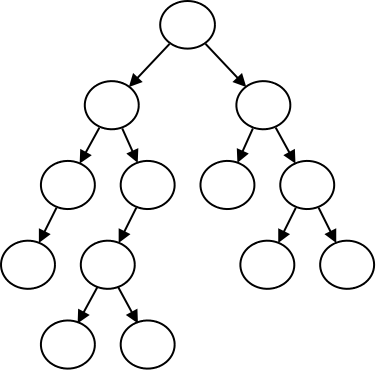When we are using binary trees inside of a program, we typically create a structure for storing the nodes:


struct Node {
type data;
Node* left;
Node* right;
};


When we did linked lists, we used a node definition similar to the above. The difference for a binary tree is how we will set up our structure. When we create nodes, we will fill in the left and right pointers such that they point to children nodes of the tree.

Note that a node can have either or both children pointers set to NULL. If both are set to NULL, than that node is a leaf.

This example demonstrates constructing a binary tree by setting the nodes up directly in code. The tree that this program constructs looks like this:### Traversals

With the data structures we have seen so far, looping through each item has been fairly easy. With an array, linked list, or any other kind of linear data structure, there are really only two ways to do, either forwards or backwards.

A tree, however, presents more of a challenge. Where should we start and where should we end? "Looping through" a tree is called traversing it. As we traverse a tree, we "visit" each of the nodes in the tree once.

There are actually three steps that we need to perform to traverse a tree:

1. Visit the root node.
2. Visit the entire left subtree.
3. Visit the entire right subtree.

Like nearly all tree algorithms, this is a recursive process. To visit the left and right subtrees, we recurse and then treat each subtree as if it was the whole tree. The base case for the traversal is when the tree we are traversing has no nodes.

There are actually different ways we can traverse the nodes based on when we visit the root node. If we do it first, we will always visit a node before visiting its children. This is called a preorder traversal.

If we instead change the traversal to visit the root node between the left and right subtrees like so:

1. Visit the entire left subtree.
2. Visit the root node.
3. Visit the entire right subtree.

This will visit the nodes in the tree in a different order. It will always visit the nodes to the left of a given node, then the root, then those to the right. This is called an inorder traversal.

Lastly, if we visit the root node last, then we will have a postorder traversal.

How can we implement these traversals in C++?


// a pre-order tree traversal
void preorder(Node* node) {
if (node != NULL) {
cout << node->data << " ";
preorder(node->left);
preorder(node->right);
}
}

// an in-order tree traversal
void inorder(Node* node) {
if (node != NULL) {
inorder(node->left);
cout << node->data << " ";
inorder(node->right);
}
}

// a post-order tree traversal
void postorder(Node* node) {
if (node != NULL) {
postorder(node->left);
postorder(node->right);
cout << node->data << " ";
}
}


### Binary Search Trees

A binary tree is a specific kind of tree, and a binary search tree is a specific kind of binary tree. Specifically it is a binary tree that obeys the following rule:

• The left subtree of a node N contains only nodes with values less than that of the node N.
• The right subtree of a node N contains only nodes with values greater than that of the node N.
• No two nodes can have the same value.

Below is an example of a binary tree that is also a binary search tree: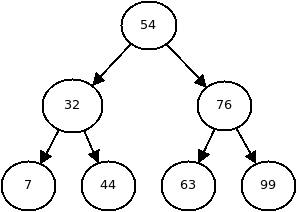The goal of binary search trees is to store data in a way that is amenable to the binary search algorithm. To search a tree for an item, we can start at the root node and compare the value there to the target. If the target is larger, then we know that the target must be in the right subtree and if the target is smaller, we know the target is in the left subtree.

Recall that with binary search we can search a sorted array in $O(\log n)$ time. We can also search a binary search tree in $O(\log n)$ time. However the advantage of a binary search tree is that we can also insert quickly.

### Inserting

If we are to insert a new value 60 into the tree above, where should it go?

We can't violate the rule that the nodes in the left subtree must be less than that of the node. So 60 must be inserted to the right side of the root node 56. Then we look at the root of the right subtree, 76. 60 is less than 76 so it must go in the left subtree of the 76 node. Then we get to the node 63, and 60 is less than that node, so it must go in the left subtree. There is no left subtree of this node, so we create one to store our new node and are done.

This is what the tree will look like after inserting 60:The algorithm to insert into a binary search tree looks like this:

1. To insert a value at a given node:
1. If the node is NULL, create a new node here with the value.
2. Otherwise, if the data at this node is less than the new value:
Insert the value in the right subtree.
3. Otherwise, if the data at this node is greater than the new value:
Insert the value in the left subtree.

How could we implement this?


void insertAt(int number, Node*& node) {
if (node == NULL) {
node = new Node;
node->data = number;
node->left = NULL;
node->right = NULL;
} else if (number < node->data) {
insertAt(number, node->left);
} else {
insertAt(number, node->right);
}
}

// insert a number calls recursive function to insert at the root
void insert(int number) {
insertAt(number, root);
}


### Balance

Note that there are multiple ways to build a binary search tree storing the same data depending on the order in which the nodes are inserted. What happens if we insert values in a sorted order?

What is produced from this is basically a singly-linked list. The reason for this is that when the nodes are inserted in order, each successive value is greater than any previous ones, so the insertion can only take the right branch. So the left branches are never used and we end up with a line of nodes: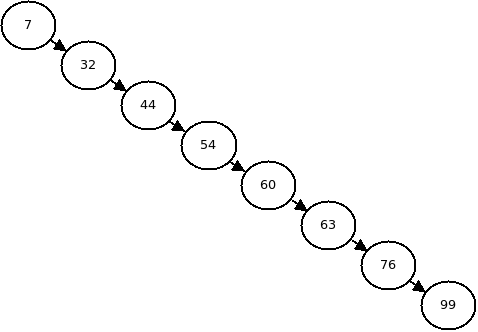This is called a degenerate tree.

The ideal case is that the tree is balanced which means that, for each node, no subtree is more than 1 node deeper than the other subtree.

### Searching

Searching a binary search tree uses the same basic idea behind regular binary search and is similar to the insertion algorithm above. At each step of the search, we consider a node and there are four possibilities. Either the node is NULL, in which case the item doesn't exist in the tree. Or the node has the data we want in which case we have found what we were searching for. Or the node has a value that is less than the item we are searching for, in which case we must search the right subtree. Or the node has a value that is more than the item we are searching for, in which case we must search the left subtree. We will start at the root and recursively work our way down.

This can be summarized in the following algorithm:

1. To search for a target at a given node:
1. If the node is NULL, return not found.
2. If the node has the data, return it.
3. If the node's value is less than target, search node->right.
4. Otherwise, search node->left.

How could we add searching into our binary tree in C++?


int* searchAt(int number, Node* node) {
if (node == NULL) {
return NULL;
} else if (number == node->data) {
return &(node->data);
} else if (number < node->data) {
return searchAt(number, node->left);
} else {
return searchAt(number, node->right);
}
}

int* search(int number) {
return searchAt(number, root);
}


### Removal

Removing a node from a binary search tree is more complicated than inserting or searching.

If we want to remove a particular element from a tree, we must first search for the node with that value (using the search described above). However, once we find the data we want to remove, there are three different cases.

##### The node is a leaf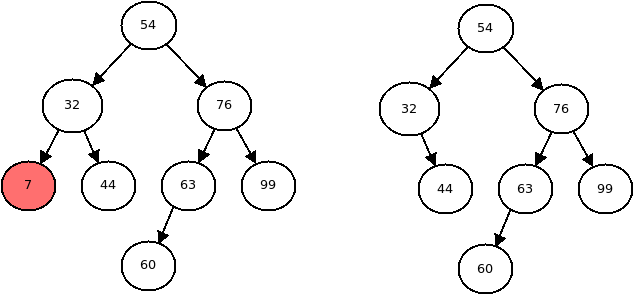In this case, we can simply remove the node, and set the link to it to NULL.

##### The node has one child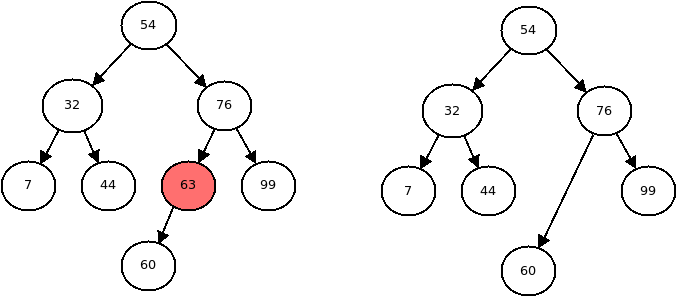In this case, we must set the parents pointer equal to the node's only child.

##### The node has two children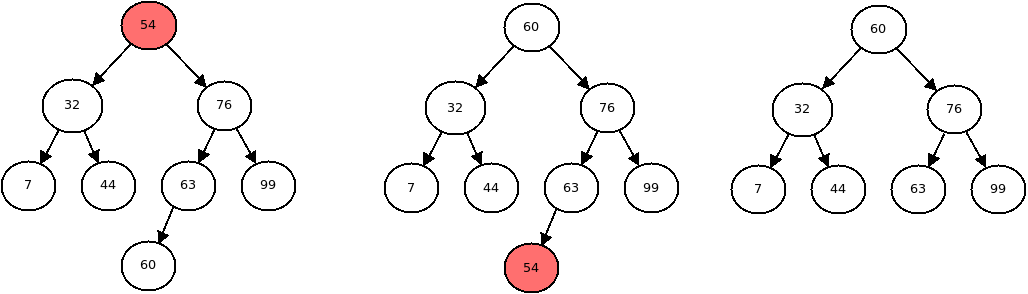This is the most complicated case.

If we just remove the node, then the tree will be split into two pieces so it will no longer be a tree. We will need to find a new root of the subtree that we are deleting. We also must obey the rules governing binary search trees, so we can't use any node for the new node.

There are only two nodes that we can use for the new root, either the largest value on the right, or the smallest value on the left.

We can then swap the data at those two nodes, as shown in the center picture above. Then there will be a duplicate node, so we must then delete that one (which we can do recursively).

The algorithm to remove a node from a binary search tree is as follows:

1. If the node has no children, delete it and set it to NULL.
2. If the node has only a left child, delete it and set it equal to node->left.
3. If the node has only a right child, delete it and set it equal to node->right.
4. If the node has two children:
1. Find the node with the least value in the node->right subtree.
2. Swap the data at the least node with the one we want to remove.
3. Remove the least node.

To find the smallest node on a subtree we can use the following algorithm:

1. If the node at the root of the subtree has no left child, return the data at the root.
2. Otherwise recursively find the smallest node on the left subtree.

This program contains all of the necessary code to remove nodes from a binary search tree.

### Balancing a Binary Search Tree

There are a few ways to balance a binary search tree. One way is to take the data out of it and store it, in sorted order, in a temporary array. Then, take the data in the array and re-insert it into the binary search tree in such a way that the tree will be balanced.

To take the data out, we can simply do an inorder traversal, and store the data in an array as we visit each node. Then we can remove each node from the tree using the remove function above repeatedly.

Then all that remains is to rebuild the tree from the array. Given a sorted array of items, in what order should we insert the items to achieve a balanced tree?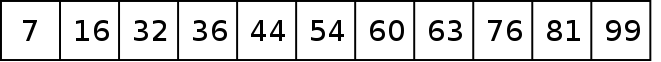In order to achieve a balanced tree, there should be a roughly equal number of nodes to the left of the root as there are to the right. Therefore the root node should be the middle item from the array. Because the first node inserted always becomes the root, we should add the middle item first.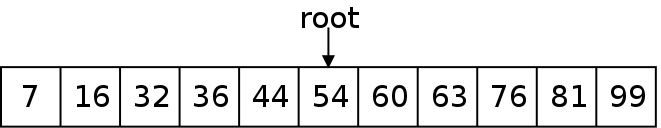After adding the root, we will have to add the items to the left and those to the right. The first item from the left we add will be the immediate left child of the root. For the same reasons that the root should be the middle item, the left child of the root should be from the middle of the left half of the array. Likewise for the right.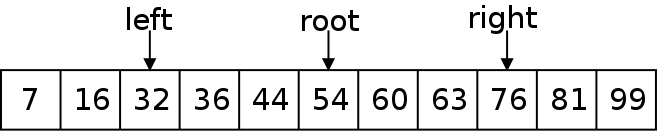We will add the middle item, then recursively add the left and right halves of the array using the same procedure. This balancing algorithm is given below:

1. Create an array to hold the elements.
2. Fill the array using an inorder traversal.
3. Remove all of the nodes from the tree.
4. Insert the entire array items using the recursive algorithm below.
5. Remove the temporary array.

This recursive algorithm will insert the array items:

1. If there are elements in the array:
1. Calculate the middle of the array.
2. Insert the middle item.
3. Recursively insert the left half.
4. Recursively insert the right half.

### Analysis

The two main binary search tree operations, insertion and searching are both $O(\log n)$ when the tree is balanced. This is due to the fact that with a perfectly balanced tree, the height is limited to the log base 2 of n and the height determines the most times we could recurse.

In the worst case, however, the tree is degenerate so the height will be equal to n. This would make insertion and searching both take $O(n)$ time.

If we balance a binary search tree, we ensure that it can be used in $O(\log n)$ time.

Assuming the tree is balanced, how do binary search trees compare with other data structures?

Arrays can also be searched in $O(\log n)$ time using binary search. However, inserting into a binary search tree is faster than inserting into an array - especially since the array must remain sorted for binary search to work.